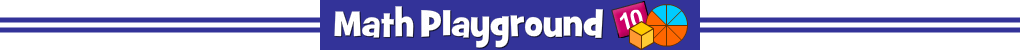The manager of the music store has misplaced the price tags.
Can you figure out the cost of each instrument?
Musical Mix-up - Learning Connections
Essential Skills
Mental Math - solving visual equations

Common Core Connection for Grades 3 and 4
Determine the unknown number in a multiplication equation relating 3 whole numbers.
Understand division as an unknown-factor problem.
Represent problems using equations with a letter standing for the unknown quantity.
More Math Games to Play